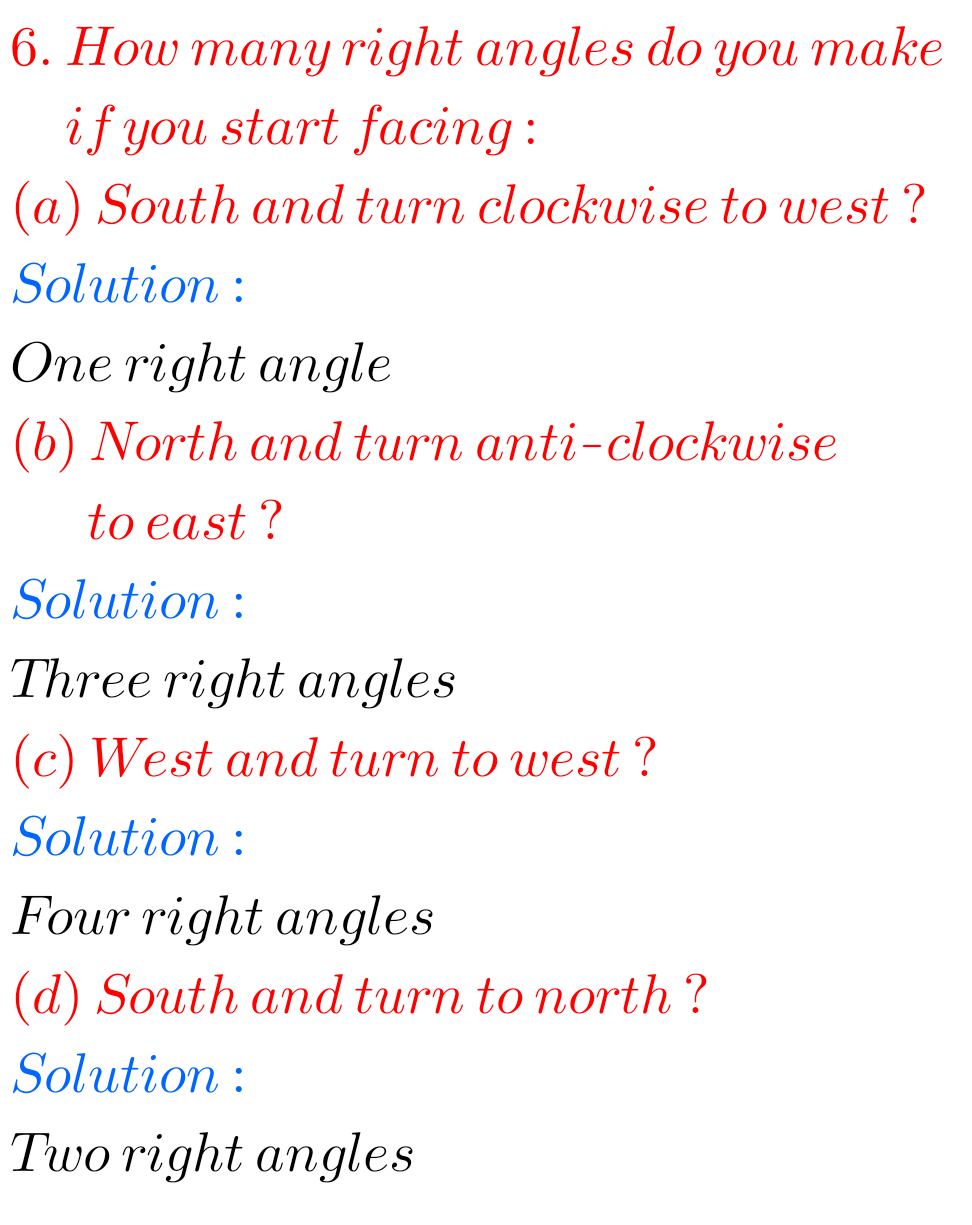## Ncert Maths Solutions for class 6 Chapter 5 exercise 5.2 solutions

Understanding Elementary Shapes Solutions chapter 5 exercise 5.2 Ncert maths class 6 Ncert Maths Class 6 chapter 5 Understanding Elementary Shapes Exercise 5.2 solutions are given. Study the textbook lesson Understanding Elementary Shapes very well. Observe the example problems and solutions given in the textbook. Observe the solutons and try them in your own method. …